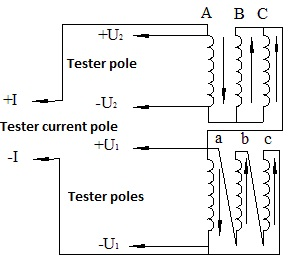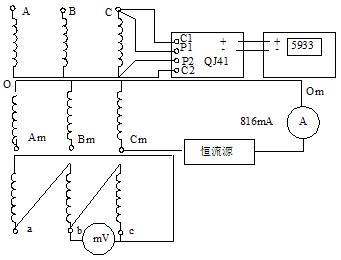Technology Literature

# How to shorten the test time of transformer winding resistance?

The voltage drop method and the bridge method are generally used. For large transformers, the inductance is very large. To speed up the measurement, a fast measurement method can be used.

Transformer winding are complex circuits that consist of distributed inductors, resistors, and capacitors. The DC winding resistance is measured by direct current between the tested terminals of the winding. After the transient process ends and the current reaches a stable state, the resistance value and the winding temperature are recorded. The key issue in measuring DC resistance is to minimize self-inductance effects. There are two ways to solve this problem.

1. Magnetic assist method

The magnetic assist method is to force the core flux to quickly become saturated, thereby reducing the self-inductance effect, which can shorten the time constant, and generally has the following methods:

(1) Use a large-capacity battery or a steady current source to measure the current.

(2) The high and low voltage windings are connected in series to measure the current, and the high voltage windings of the same phase and the same polarity are used for magnetic fluxing. Since the number of turns of the high voltage winding is much higher than that of the low voltage, the core can be saturated with a small current by means of the number of ampere turns of the high voltage winding.

(3) A DC resistance tester using a constant voltage constant current source method. When used, the high and low voltage windings can be connected in series, and the dual channel is used to measure the high and low voltage windings at the same time, which better solves the difficulty of the DC resistance test of the three-phase five-column large-capacity transformer. Generally test a 360MVA, 500kV or 220kV transformer winding DC resistance takes about 30 ~ 40min, the measurement wiring shown in Figure 1-1.2. Degaussing method

The degaussing method is opposite to the magnetically assisted method in that the magnetic flux passing through the core is made zero. There are two ways to use it:

(1) Zero-sequence impedance method. This method is only applicable to transformers with a three-column core Y-N connection. The three-phase windings are connected in parallel and energized at the same time. Since the magnetic flux needs to be closed by the air gap, the magnetic resistance of the magnetic circuit is greatly increased, and the inductance of the winding is reduced, so that the time for measuring the resistance is shortened.

(2) Magnetomotive force cancellation method. In the test, in addition to the current flowing through the measured winding, the current is also passed through the non-measured winding, so that the magnetomotive force generated by the two is equal in magnitude and opposite in direction, and cancel each other, thereby keeping the magnetic flux in the core close to zero. The inductance of the winding is minimized for the purpose of shortening the measurement time. The measurement wiring is shown in Figure 1-2.